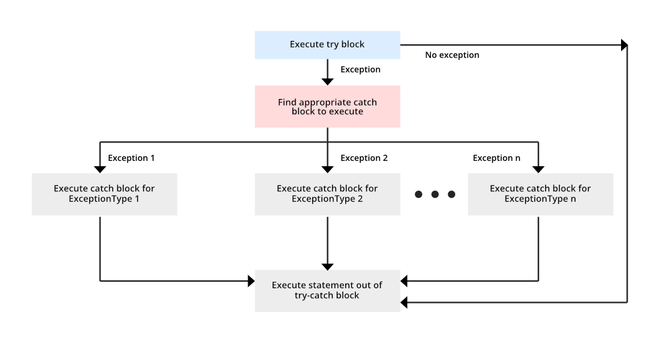Open in App
Not now

# Java Multiple Catch Block

• Difficulty Level : Easy
• Last Updated : 07 Dec, 2021

Before Java 7, we had to catch only one exception type in each catch block. So, whenever we needed to handle more than one specific exception but take some action for all exceptions, we had to have more than one catch block containing the same code.

In the following code, we have to handle two different exceptions but take the same action for both. So we needed to have two different catch blocks as of Java 6.0.

## Java

 `// A Java program to demonstrate that we needed` `// multiple catch blocks for multiple exceptions` `// prior to Java 7`   `import` `java.util.Scanner;` `public` `class` `Test` `{` `    ``public` `static` `void` `main(String args[])` `    ``{` `        ``Scanner scn = ``new` `Scanner(System.in);` `        ``try` `        ``{` `            ``int` `n = Integer.parseInt(scn.nextLine());` `          `  `            ``if` `(``99``%n == ``0``)` `                ``System.out.println(n + ``" is a factor of 99"``);` `        ``}` `        ``catch` `(ArithmeticException ex)` `        ``{` `            ``System.out.println(``"Arithmetic "` `+ ex);` `        ``}` `        ``catch` `(NumberFormatException ex)` `        ``{` `            ``System.out.println(``"Number Format Exception "` `+ ex);` `        ``}` `    ``}` `}`

Input 1:

`GeeksforGeeks`

Output 1:

```Exception encountered java.lang.NumberFormatException:

For input string: "GeeksforGeeks"```

Input 2:

`0`

Output 2:

`Arithmetic Exception encountered java.lang.ArithmeticException: / by zero`

### Multiple Catch Block in Java

Starting from Java 7.0, it is possible for a single catch block to catch multiple exceptions by separating each with | (pipe symbol) in the catch block.

Catching multiple exceptions in a single catch block reduces code duplication and increases efficiency. The bytecode generated while compiling this program will be smaller than the program having multiple catch blocks as there is no code redundancy.

Note: If a catch block handles multiple exceptions, the catch parameter is implicitly final. This means we cannot assign any values to catch parameters.

Syntax:

```try {
// code
}
catch (ExceptionType1 | Exceptiontype2 ex){
// catch block
}```

### Flow Chart of Java Multiple Catch Block## Java

 `// A Java program to demonstrate` `// multicatch feature`   `import` `java.util.Scanner;`   `public` `class` `Test` `{` `    ``public` `static` `void` `main(String args[])` `    ``{` `        ``Scanner scn = ``new` `Scanner(System.in);` `        ``try` `        ``{` `            ``int` `n = Integer.parseInt(scn.nextLine());` `            ``if` `(``99``%n == ``0``)` `                ``System.out.println(n + ``" is a factor of 99"``);` `        ``}` `        ``catch` `(NumberFormatException | ArithmeticException ex)` `        ``{` `            ``System.out.println(``"Exception encountered "` `+ ex);` `        ``}` `    ``}` `}`

Input 1:

`GeeksforGeeks`

Output 1:

```Exception encountered java.lang.NumberFormatException:

For input string: "GeeksforGeeks"```

Input 2:

`0`

Output 2:

```Exception encountered

java.lang.ArithmeticException: / by zero```

A catch block that handles multiple exception types creates no duplication in the bytecode generated by the compiler. That is, the bytecode has no replication of exception handlers.

### Important Points:

1. If all the exceptions belong to the same class hierarchy, we should be catching the base exception type. However, to catch each exception, it needs to be done separately in their catch blocks.

2. Single catch block can handle more than one type of exception. However, the base (or ancestor) class and subclass (or descendant) exceptions can not be caught in one statement. For Example

```// Not Valid as Exception is an ancestor of
// NumberFormatException
catch(NumberFormatException | Exception ex) ```

3. All the exceptions must be separated by vertical bar pipe |.